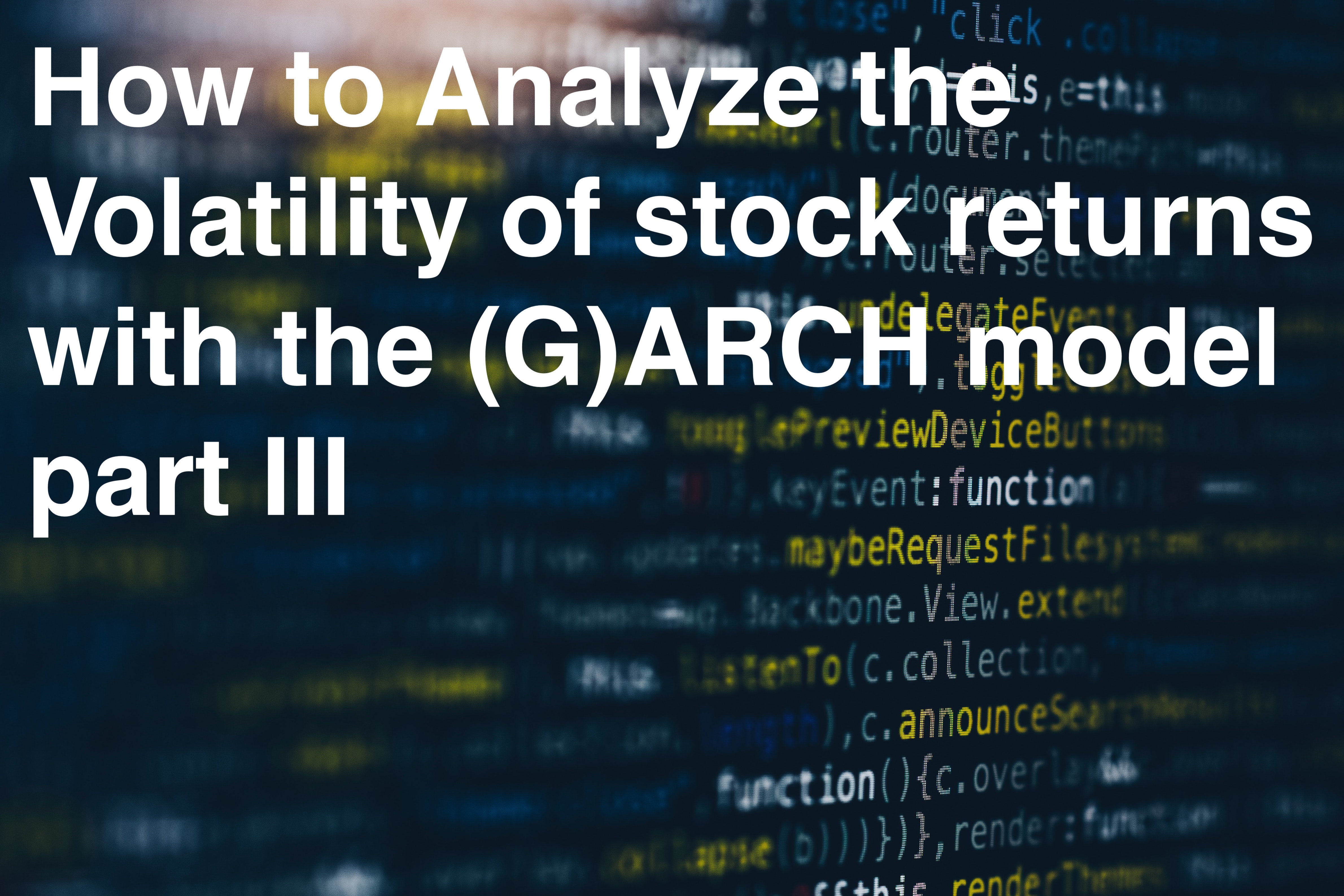10
min

# How to Analyze the Volatility of stock returns with the (G)ARCH model part III

Presentation of the ACF (autocorrelation function) and PACF (Partial autocorrelation function) results## 4.2 Autocorrelation-Analysis

### 4.2.1 ACF (autocorrelation function)

The time inconsistencies of volatilities can be seen in the observation of returns in Figures1.1 and 1.2 (Schmelzer 2009: 18). It can be clearly seen that large and small returns tend to follow large and small price changes. This pattern is called a volatility cluster(Mandelbrot/Variation 1963: 394ff). To analyze the autocorrelation and to proceed with the analysis, two functions are suitable. On the one hand the AKF: the autocorrelation function, and the PAKF: the partial autocorrelation function. Autocorrelation describes the correlation of a function or signal with itself at an earlier point in time in the time series (Stock/Watson2019: 558).

Figures 1.4 and 1.5 show the autocorrelation functions for the Nasdaq100 and gold log returns. The autocorrelation function describes the correlation between the current point in time Y(t) and an earlier point in time in the time series. For example, the first lag measures the correlation between Y(t) and Y(t-1) (Stock/Watson 2019: 558). The blue dashed lines represent a significant threshold. Any interval that exceeds the blue dashed line is significant(Kosfeld 2007: 1). You can see a clear difference between the Nasdaq100 and gold here.While several lags are significant for the Nasdaq100, for gold it can be seen that hardly any lag has a significant impact other than lag 17. This is also consistent with the first observation of log returns, which shows that the Nasdaq100 fluctuates significantly more are compared to gold.

### 4.2.2 PACF (Partial autocorrelation function)

As a next step, the analysis of the partial autocorrelation function can be helpful. The PAKF measures the linear relationship between Y(t) and Y(t+k) while eliminating the influence of the intervening variables (Kosfeld 2007: 1). Figures 1.6 and 1.7 show the PAKF for Nasdaq100 and gold. As with the AKF, it can be seen that gold has significantly less significant lags than the Nasdaq100.

In Figure 1.6, it can be seen that there is a high significance in the first lags up to the ninth lag. After this lag, the significance is significantly lower. Still, you can see significant outliers at lag 22 and lag 26. For gold, it's clear that only lag 17 and lag 29 are significant. The scaling of the Y-axis is also interesting, since we see a scaling of [-0.10 - 0.05] for the Nasdaq100 and a scaling of [-0.04 - 0.04] for gold. This ties in with the previous finding that the Nasdaq100 isa lot more volatile and therefore has a lot more significant points than gold. Gold has a very concentrated swing around zero.

if you want to continue reading go to part IV

#### Sources:

Marcus Schmelzer, (2009), The volatility of financial market data, theoretical foundations and empirical analyzes of hourly return time series and risk measures, Universität Köln